Courses

# Revisal Problems (Past 13 Year) JEE Main (Coordination Compounds)

## 29 Questions MCQ Test | Revisal Problems (Past 13 Year) JEE Main (Coordination Compounds)

Description
This mock test of Revisal Problems (Past 13 Year) JEE Main (Coordination Compounds) for Class 12 helps you for every Class 12 entrance exam. This contains 29 Multiple Choice Questions for Class 12 Revisal Problems (Past 13 Year) JEE Main (Coordination Compounds) (mcq) to study with solutions a complete question bank. The solved questions answers in this Revisal Problems (Past 13 Year) JEE Main (Coordination Compounds) quiz give you a good mix of easy questions and tough questions. Class 12 students definitely take this Revisal Problems (Past 13 Year) JEE Main (Coordination Compounds) exercise for a better result in the exam. You can find other Revisal Problems (Past 13 Year) JEE Main (Coordination Compounds) extra questions, long questions & short questions for Class 12 on EduRev as well by searching above.
QUESTION: 1

### Only One Option Correct Type Direction (Q. Nos. 1-28) This section contains 28 multiple choice questions. Each question has four choices (a), (b), (c) and (d), out of which ONLY ONE option is correct. Q. Which has higher van’t Hoff factor value in aqueous solution?

Solution:

One molecule of potash alum produces 8 ions in solution (K2SO4.AI2(SO4)3.24H2O)
One molecule of Mohr's salt produces 5 ions in solution
(FeSO4).(NH4)2SO4.6H2O) One molecule of potassium ferrocyanide produces 5 ions in solution [K4[Fe(CN)6].
One molecule of carnalite produces 5 ions in solution (KCI-MgCI2.6H2O)

QUESTION: 2

### A chelating ligand has two or more than two donor atoms to bind a single metal ion. Which of the following is not a chelating agent?

Solution:

Thiosulphate is not a chelating agent.

QUESTION: 3

### Pick up a poor electrolytic conductor complex in solution,

Solution:

[Co(NH3)3(NO2)3] complex is poor electrolytic conductor.

QUESTION: 4

How many EDTA molecules are required to make an octahedral complex with a Ca2+ ion?

Solution:

EDTA is a hexadentate ligand. Its coordination number is Six

QUESTION: 5

Which of the following is an outer orbital complex?

Solution:

Nickel complexes with coordination number six are always outer complexes with Ni (sp3d2) hybridisation.

QUESTION: 6

The structure of the complexes  [Cu(NH3)4] (ClO4) and  [Cu(NH3)4]ClO4  in solution respectively are

Solution:

In the complex [Cu(NH3)4](CIO4), copper has dsp2 (square planar) while in complex [Cu(NH3)4]CIO4, copper has sp3 (tetrahedral) hybridisation.

QUESTION: 7

EAN value of the complex is not equal to 36 in

Solution:

When EAN calcula tion is done for [Ni(H2O)6]2+, i.e. [Z-oxidation number) + 2 x (coordination number)], we get 38. For all others we get 36.

QUESTION: 8

The correct statement is

Solution:

[MnBr4]2- is tetrahedral complex.

QUESTION: 9

How many moles of AgCl would be obtained from when 100 moles of 0.1 M CoCl3. 5NH3 is treated with eccess AgNO3?

Solution:

100 mL 0.1 M complex = 0.01 moles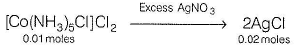QUESTION: 10

The IUPAC name of  [Ni(NH3)4][NiCl4]

Solution:

Tetramminenickel (II) tetrachloronickelate (II)

QUESTION: 11

Which is the correct?

Solution:

[Co(en)3]CI3 shows only optical isomerism.

QUESTION: 12

Extent of crystal field splitting in octahedral complexes of the given metal with particular weak field ligand are such that

Solution:

Fe(iii) < Cr(iii) < Rh(iii) < Ir(iii)

QUESTION: 13

Which of the following is correct arrangement of ligands in terms of the Dq values of their complexes with any particular 'hard' metal ion?

Solution:

Cl- < F- <  NCS-< NH< CN-

QUESTION: 14

Cr3+ form four complexes with four different ligands which are [Cr(CI)6]3- , [Cr(H2O)6]3+, [Cr(NH3)6]3+ and [Cr(CN)6]3- . The order of CFSE (Do) in these com ple xes is in the order

Solution:

[CrCI6]3- > [Cr(H2O)6]3+ > [Cr(NH3)6]3+ > [Cr(CN)6]3-

QUESTION: 15

The IUPAC name of [Fe(O2)(CN)4Cl]-4 is

Solution:

Chlorotetracyano superoxoferrate (II) ion

QUESTION: 16

The value of 'spin only' magnetic moment for one of the following configuration in octahedral field is 3.87 BM. The correct one is

Solution:

Magnetic moment 3.87 BM means it has three unpaired electrons. This is possible in d(in weak as well as strong field ligand)

QUESTION: 17

There are four complexes of Ni. Select the complex(es) which will be attracted by magnetic field.

I. [Ni(CN)4]2- II.[NiCl4]2- III.Ni(CO)IV. [Ni(H2O)6]2+

Solution:

Diamagnetic complexes are [Ni(CN)4]2- and Ni(CO)4.
Paramagnetic complexes are
[NiCI4]2- and [Ni(H2O)6]2+ (attracted)

QUESTION: 18

An octahedral com plex with m olecular form ula 5NH3.Cl.SO4 has two isomers A and B. The solution of A gives a white precipitate with AgNO3 solution and the solution of B gives white precipitate with BaCI2 solution .The type of isomerism exhibited by the complex is

Solution: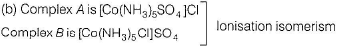QUESTION: 19

Both geometrical and optical isomerism are shown by

Solution:

[Co(en)2CI2]+ this has cis and trans isomers.
The cis isomer exists as enantiomeric pair .Hence, total isomers are 3.

QUESTION: 20

Low spin complex of d6 cation in an octahedral field will have which of the following energy?

Solution:

CFSE = - (0.4 x 6 Δo + 3P)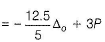(∴ P means pairing energy which is the energy required to pair two electrons against electron-electron repulsion in the same orbital)

QUESTION: 21

The crystal field stabilisation energy(CFSE) and the spin only magnetic moment in Bohr magneton (BM) for the complex K3[Fe(CN)6] respectively, are

Solution:

In K3[Fe(CN)6], iron is in +3 oxidation state.
∴ In strong ligand field, configuration is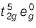.  Hence, CFSE is -5 x 0.4 = -2,0 Δo.
Since, it has one unpaired electron, magnetic moment is √3 BM.

QUESTION: 22

If the bond length of CO bond in carbon monoxide is 1.128 A°,then what is the value of CO bond length in Fe(CO)5?

Solution:

Due to synergic effect, CO bond order decreases and bond length increases.

QUESTION: 23

Crystal field theory does not explain which of the following property coordination compounds?

Solution:

The covalent character of the bond between metal and ligand

QUESTION: 24

The correct order of ligand field strength is

Solution:

Cl < H2O < NH< CO

QUESTION: 25

What is(are) number of unpaired electron(s) in the square planer [Pt(CN)4]2- ions?

Solution:

The number of unpaired electrons in the square planar [Pt(CN)4]2- is zero.

QUESTION: 26

In decacarbonyl dimanganese (0), the number of CO groups bonded in between the Mn atoms are

Solution:

In decacarbonyl dimanganese (0), the number of CO group bonded in between the Mn atoms are shown in the following figure.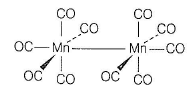QUESTION: 27

The ligand that shows synergic effect is

Solution:

CO is a ligand that forms sigma bond with metal by donating electrons and pi bond by accepting electrons from the central metal ion.
This is called synergic effect.

QUESTION: 28

For the reactions and their equilibrium constants given below: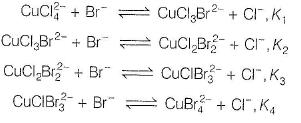Q.

The equilibrium constant, K for the reaction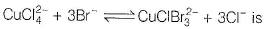Solution:

When an equation is obtained by adding two or more equations the new equilibrium constant is the product of the equilibrium constants of the added equations.
Therefore, K = K1 x K2 x K3.

QUESTION: 29

Statement Type

Direction (Q. No. 29) This section is based on Statement I and Statement II. Select the correct answer from the codes given below.

Q.

Statement I : [Fe(CN)6]3- ion shows magnetic moment corresponding to two unpaired electrons.

Statement lI : Because it has d2sp3 type hybridisation.

Solution:

Iron atom (Z = 26) has electronic configuration 3d64s2. In this complex iron is in +3 oxidation state and has electronic configuration 3d5.
It has been experimentally observed that the complex has one unpaired electron.